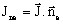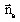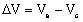# Definition of a polarization law

## Introduction

A polarization law is a law that connects the difference of electric potential and the current density at the level of an electrochemical interface (electrode/electrolyte).

## Polarization law

A polarization law is defined by a series of values: (normal component of the current density Jne / difference ΔV of electric potential)

Current density:

The normal component of the current density at a point () is considered positive when the current passes from the electrolyte towards the cathode. The normal unit vectoris directed from the electrolyte towards the cathode.

Difference of electric potential:

The difference ∆V of the electric potential represents the electric potential of the electrolyte at a point very close to the cathode, when that of the cathode is null:where:

• Ve is the electric potential of the electrolyte
• Vc is the electric potential of the cathode## Definition of a polarization law

The values of the normal component of the current density Jne must be strictly increasing.

The values of the differences ΔV of the electric potential must be: either strictly increasing, or strictly decreasing.

## Modes of definition

You can define a polarization law in two different ways:

• directly: defining the couple of values in a dialog box
• indirectly: defining the couple of values in a text file

The file text must meet the following requirements :

• only two values per line, separated by a space
• no title